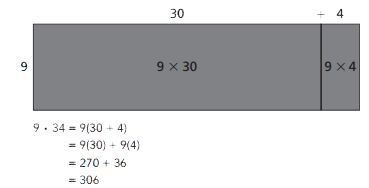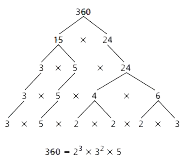Toggle Accessibility Tools

# 6-1 Prime Time - Concepts and Explanations

## Order of Operations

The universally agreed upon order for solving math problems. The acronym PEMDAS is used to help remember the order of the steps.

### Example

1. Compute any expression with parentheses.
2. Compute any exponent.
3. Do all multiplication and division in order from left to right.
4. Do all addition and subtraction in order from left to right.

(4 + 6) x 2 = (10) x 2
= 20

## Distributive Property

The Distributive Property shows how a number can be written as two equivalent expressions. A number can be expressed as both a product and a sum. Multiplication is distributed over addition. It can be helpful for understanding the structure of multidigit multiplication.

### Example## Prime

A number with exactly two factors, 1 and the number itself.

### Example

Examples of primes are 11, 17, 53, and 101. The number 1 is not a prime number, since it has only one factor.

All of the factors of 11 are 1 and 11. All of the factors of 17 are 1 and 17.

## Composite

A whole number with factors other than itself and 1 or a whole number that is not prime.

### Example

Some composite numbers are 6, 12, 20, and 1,001. Each of these numbers has more than two factors.

All of the factors of 6 are 1, 2, 3, 6. All of the factors of 1,001 are 1, 7, 11, 13, 77, 91, 142 and 1001.

## Common Multiples

A multiple that two or more numbers share. The least common multiple (LCM) of 12 and 18 is 36.

### Example

The first few multiples of 5 are 5, 10, 15, 20, 25, 30, 35, 40, 45, 50, 55, 60, 65, and 70.

The first few multiples of 7 are 7, 14, 21, 28, 35, 42, 49, 56, 63, 70, 77, 84, and 91.

From these lists you can see that two common multiples of 5 and 7 are 35 and 70. There are more common multiples that can be found.

## Common Factors

A factor that two or more numbers share. The greatest common factor (CGF) of 12 and 18 is 6.

### Example

The number 7 is a common factor of 14 and 35 because 7 is a factor of 14 (14 = 7 x 2) and 7 is a factor of 35 (35 = 7 x 5).

## Prime Factorization

A product of prime numbers, resulting in the desired number. The prime factorization of a number is unique except for the order of the factors. This is the Fundamental Theorem of Arithmetic.

### ExampleThe prime factorization of 360 is 2 x 2 x2 x3 x5.

Although you can switch the order of the factors, every prime product string for 360 will have three 2s, two 3s and one 5.